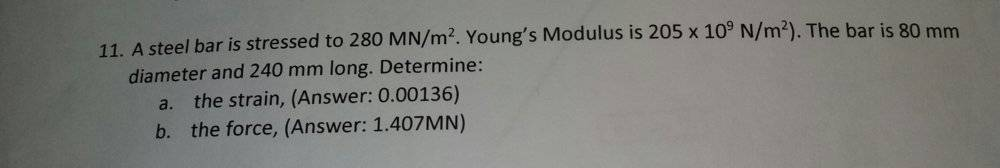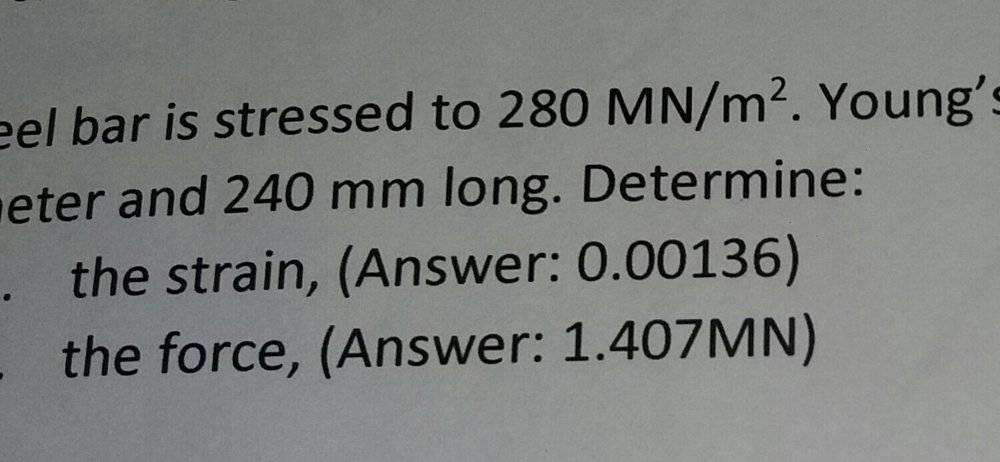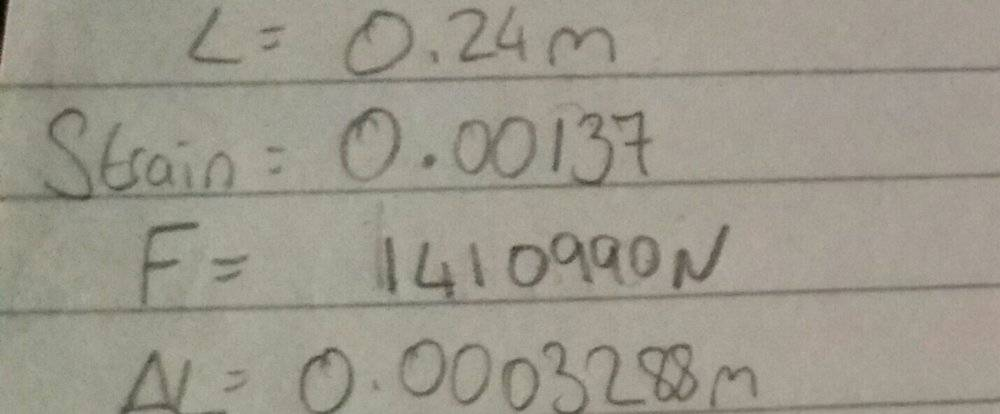# Young's modulus: Stress, Strain and Force for a Steel Bar

Homework Statement:
Problem to find strain and force
Relevant Equations:
F = A E delta L
L
As all attempts to get it right but without success this is one of the problems with my workout . Where i did wrong calculations ?
The questions got the answers in brackets.#### Attachments

Last edited by a moderator:

Chestermiller
Mentor
It looks like your answers for the strain and force match the book answers. What's the problem?

•chriscarson and etotheipi
I know that can be rounded or something but when they have different numbers I need to be sure

Chestermiller
Mentor
I know that can be rounded or something but when they have different numbers I need to be sure
What’s different?Strain and force have different numbers you can see .

etotheipi
This is just because of rounding errors. Either yourself or the author have prematurely truncated the results from previous steps.

This goes to show the benefit of working algebraically and only substituting in numerical values in the final line of working. The alternative is to use the STORE buttons on your calculator, which is much more fiddly...

•chriscarson
This is just because of rounding errors. Either yourself or the author have prematurely truncated the results from previous steps.

This goes to show the benefit of working algebraically and only substituting in numerical values in the final line of working. The alternative is to use the STORE buttons on your calculator, which is much more fiddly...

The strain maybe close but the force is way out i think.

Chestermiller
Mentor
When you multiplied the stress by the area to get the force, you must have made a mistake in arithmetic. When I use your calculated area, I get their force. When I divide the stress by Young's modulus to get the strain, I confirm your value of 0.00137.

•chriscarson
When you multiplied the stress by the area to get the force, you must have made a mistake in arithmetic. When I use your calculated area, I get their force. When I divide the stress by Young's modulus to get the strain, I confirm your value of 0.00137.

So for the force you just multiplied stress with by area ?
If you have time you can work all the problem because I showed it .

we also had a formula F = k delta L , is that another way to find the F ? the only problem is what is the k .

Last edited:
So for the force you just multiplied stress with by area ? I multiplied area, young modulus and delta L over lenght
If you have time you can work all the problem because I showed it .